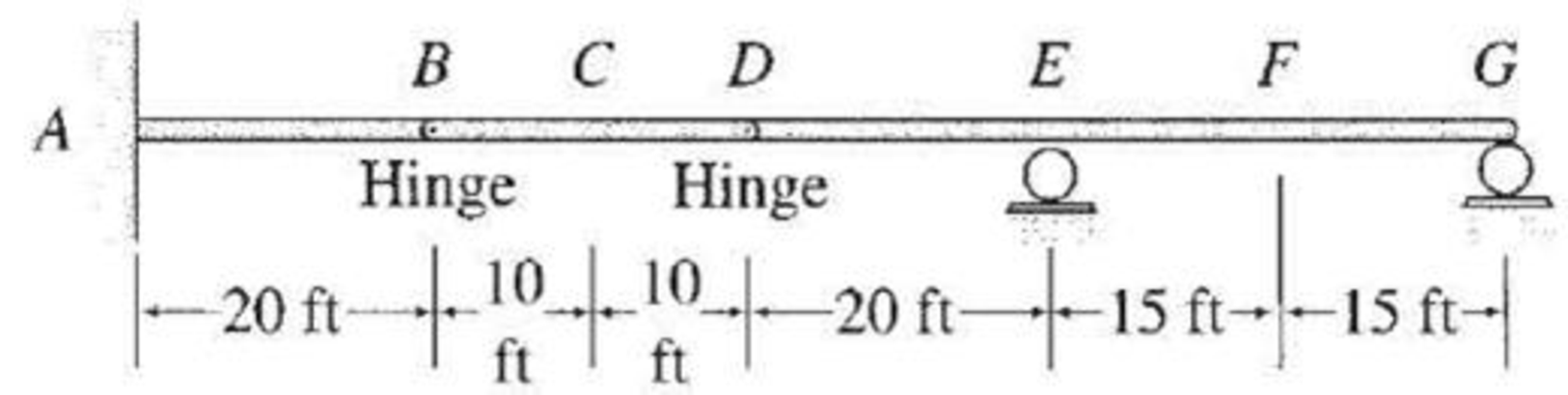# Draw the influence lines for the shears and bending moments at points C and F of the beam shown in Fig. P8.29. FIG. P8.29, P8.30

#### Solutions

Chapter
Section
Chapter 8, Problem 30P
Textbook Problem
23 views

## Draw the influence lines for the shears and bending moments at points C and F of the beam shown in Fig. P8.29.FIG. P8.29, P8.30

To determine

Draw the influence lines for the shear and bending moment at point C and F.

### Explanation of Solution

Calculation:

Use Muller-Breslau's principle.

Influence line ordinate for shear at C.

Cut the beam at C to obtain the released structure of the beam. Next apply a small relative displacement in positive direction of SC by moving end C of the portion BC downward by Δ1 and end C of the portion CD upward by Δ2 to obtain the deflected shape.

Sketch the deflected shape of the released beam when the beam cut at C as shown in Figure 1.

The general shape of the influence line for shear (SC) at C is the similar of deflected shape.

Sketch the general shape of the influence line for shear (SC) at C as shown in Figure 2.

Find the numerical value of influence line ordinate of SC at C.

Place the 1 k load first just to the left of C and then just to the right of C.

Sketch the free body diagram of beam as shown in Figure 3.

Find the reaction (R) at hinge B and D.

The point C is the center of support B and D. Hence, the reaction on the hinge B and D is half of the load. Then,

R=12k

Find the shear at C(SC,L) when unit load 1 k placed just left of C using the equation:

SC,L=R

Substitute 12k for R.

SC,L=12k

Thus, the value of influence line ordinate just left of C is 12k/k.

Find the shear at F(SC,R) when unit load 1 k placed just right of C using the equation:

SC,R=R

Substitute 12k for R.

SC,R=12k

Thus, the value of influence line ordinate just right of C is 12k/k.

The numerical value of influence line ordinate in different points on the beam for shear SC are determined using geometry (similar triangle) of the influence line and summarize the values as in Table 1.

 x (ft) Points Influence line ordinate of SC(k/k) 0 A 0 20 B 0 30 C− −12 30 C+ 12 40 D 0 60 E 0 75 F 0 90 G 0

Sketch the influence line diagram for shear at point C as shown in Figure 4.

Influence line ordinate for moment at C.

Sketch the deflected shape of the released beam when insert a hinge at C as shown in Figure 5.

Insert a hinge at C to obtain the released structure of the beam. Next apply a small rotation θ in positive direction of MC at C in the released structure. The rotation θ rotate the portion BC in counterclockwise and the portion CD in clockwise to obtain the deflected shape.

The general shape of the influence line for bending moment (MC) at C is the similar of deflected shape.

Sketch the general shape of the influence line for bending moment (MC) at C as shown in Figure 6.

Find the numerical value of influence line ordinate for MC at C.

Refer Figure 3.

Find the moment at C from B.

Consider clockwise moment as positive and anticlockwise moment as negative.

MC=R(10)=12(10)=5k-ft

Thus, the value of influence line ordinate of MC at C is 5k-ft/k.

The remaining values of influence line ordinate are determined by geometry (similar triangle) of the influence line and summarize the values as in Table 2.

 x (ft) Points Influence line ordinate of MC(k-ft/k) 0 A 0 20 B 0 30 C 5 40 D 0 60 E 0 75 F 0 90 G 0

Sketch the influence line diagram for moment at point C as shown in Figure 7.

Influence line ordinate for shear at F.

Cut the beam at F to obtain the released structure of the beam. Next apply a small relative displacement in positive direction of SF by moving end F of the portion EF downward by Δ1 and end F of the portion FG upward by Δ2 to obtain the deflected shape

### Still sussing out bartleby?

Check out a sample textbook solution.

See a sample solution

#### The Solution to Your Study Problems

Bartleby provides explanations to thousands of textbook problems written by our experts, many with advanced degrees!

Get Started

Find more solutions based on key concepts
For problems 15.33 through 15.40, go through each line of MATLAB code and show the result, or indicate if an er...

Engineering Fundamentals: An Introduction to Engineering (MindTap Course List)

Define the term, digital literacy.

Enhanced Discovering Computers 2017 (Shelly Cashman Series) (MindTap Course List)

What is the smallest graduation on an inch micrometer with a vernier scale?

Precision Machining Technology (MindTap Course List)

Solve the preceding problem for the element shown in the figure.

Mechanics of Materials (MindTap Course List)

If your motherboard supports ECC DDR3 memory, can you substitute non-ECC DDR3 memory?

A+ Guide to Hardware (Standalone Book) (MindTap Course List)